Chapter 1: Algebra Review

# 1.4 Properties of Algebra (Review)

When doing algebra, it is common not to know the value of the variables. In this case, simplify where possible and leave any unknown variables in the final solution. One way to simplify expressions is to combine like terms.

Like terms are terms whose variables match exactly, exponents included. Examples of like terms would be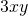and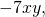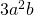and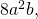or −3 and 5. To combine like terms, add (or subtract) the numbers in front of the variables and keep the variables the same.

Example 1.4.1

Simplify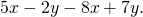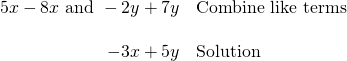Example 1.4.2

Simplify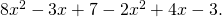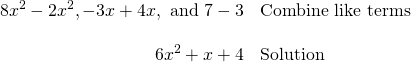When combining like terms, subtraction signs must be interpreted as part of the terms they precede. This means that the term following a subtraction sign should be treated like a negative term. The sign always stays with the term.

Another method to simplify is known as distributing. Sometimes, when working with problems, there will be a set of parentheses that makes solving a problem difficult, if not impossible. To get rid of these unwanted parentheses, use the distributive property and multiply the number in front of the parentheses by each term inside.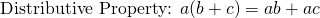Several examples of using the distributive property are given below.

Example 1.4.3

Simplify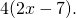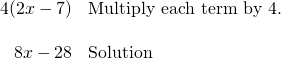Example 1.4.4

Simplify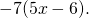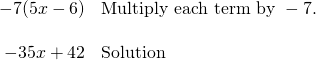In the previous example, it is necessary to again use the fact that the sign goes with the number. This means −6 is treated as a negative number, which gives (−7)(−6) = 42, a positive number. The most common error in distributing is a sign error. Be very careful with signs! It is possible to distribute just a negative throughout parentheses. If there is a negative sign in front of parentheses, think of it like a −1 in front and distribute it throughout.

Example 1.4.5

Simplify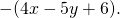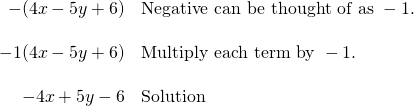Distributing throughout parentheses and combining like terms can be combined into one problem. Order of operations says to multiply (distribute) first, then add or subtract (combine like terms). Thus, do each problem in two steps: distribute, then combine.

Example 1.4.6

Simplify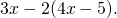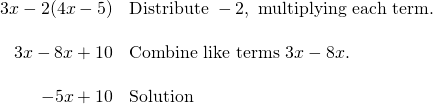Example 1.4.7

Simplify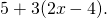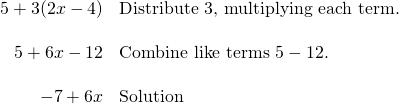In Example 1.4.6, −2 is distributed, not just 2. This is because a number being subtracted must always be treated like it has a negative sign attached to it. This makes a big difference, for in that example, when the −5 inside the parentheses is multiplied by −2, the result is a positive number. More involved examples of distributing and combining like terms follow.

Example 1.4.8

Simplify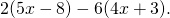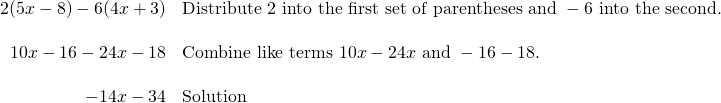Example 1.4.9

Simplify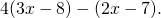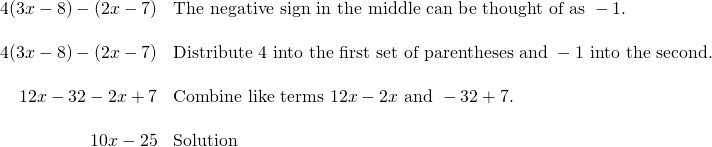# Questions

For questions 1 to 28, reduce and combine like terms.

1.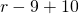2.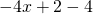3.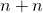4.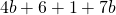5.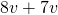6.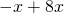7.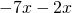8.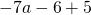9.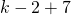10.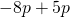11.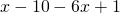12.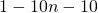13.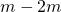14.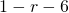15.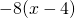16.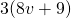17.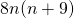18.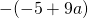19.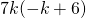20.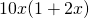21.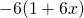22.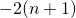23.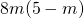24.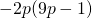25.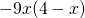26.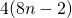27.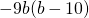28.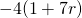For questions 29 to 58, simplify each expression.

1.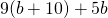2.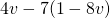3.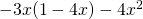4.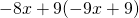5.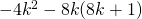6.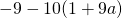7.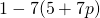8.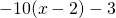9.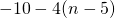10.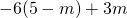11.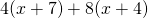12.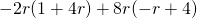13.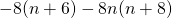14.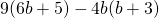15.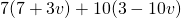16.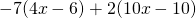17.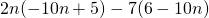18.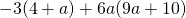19.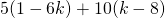20.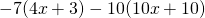21.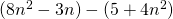22.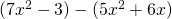23.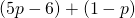24.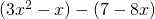25.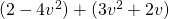26.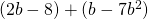27.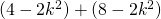28.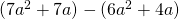29.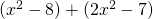30.